# Modal test

Modal test is a process to determine the transfer characteristic of a system. It is most often used to find the natural frequencies of a mechanical structure using hammer or shaker excitations.

• Required hardware: Sirius, Dewe43
• Required software: Dewesoft DSA or SE, PROF and FRF option

## Modal test (introduction)

Modal test is a mathematical module that allows us to calculate the transfer function ($H$) between two signals.

$$H = \frac{\text{Output}}{\text{Input}}$$

Input and Output signals are usually mechanical or electrical nature. Mechanical means exciting structure with hammer or shaker and measure response with an accelerometer. Electrical can be for example input and output voltage of the amplifier in order to get transfer function of the amplifier.

Transfer function ($H$) is a relation between two signals in a complex form (real and imaginary vs frequency).

## Data collection:

Calculations can be done in three ways:

## Setup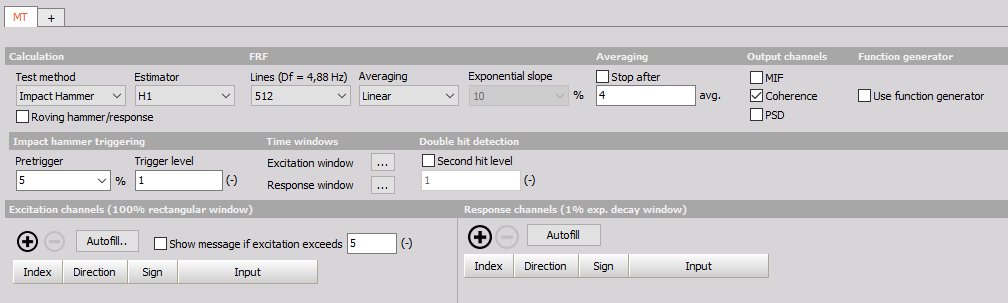### Calculation

Transfer function is calculated via the standard Frequency response function algorithm or FRF for short. It is possible to calculate all excitation/response pair at once or in groups.

#### Test method

• Impact Hammer (Triggered acquisition) - In triggered acquisition, blocks of data are taken when trigger level is exceeded.
• Shaker (Free run) - In Free run blocks of data are taken continuously (one following another with overlap if specified).
• ODS (Operational Deflection  Shapes) -  A single accelerometer is used as means of excitation measurement, others are used for the response. This method is used if excitation by hammer or shaker is not an option.

Use one excitation channel and several response channels or several excitation channels and one response channel in a single measurement.

Do multiple measurements with one or more excitation points to measure one or more responses.

#### FRF estimator

• H1 - The most commonly used estimator is the H1-estimator, which assumes that there is no noise on the input and consequently that all the input measurements are accurate. All noise is assumed to be on the output.
• H2 - Alternatively, the H2 estimator can be used. This assumes that there is no noise on the output and consequently that all the output measurements are accurate. Noise is assumed to be only on input.

### FRF (frequency response function)

• Lines - The number of lines for FFT (block size equals two times lines).
• Averaging type - Select the averaging type from Linear, Peak hold, Exponential
• Exponential slope - Define slope falloff in per cent if Exponential is used
• Stop after - Stop after the set number of averages

### Stop after

Select the check-box to enable Averaging and input the maximum number of measurements. This will enable you to take the predefined number of measurements and average them for a combined result. If the number of averages is not reached, the result will be calculated from the completed measurements. After the number is reached, you will not be able to do additional measurements for that group.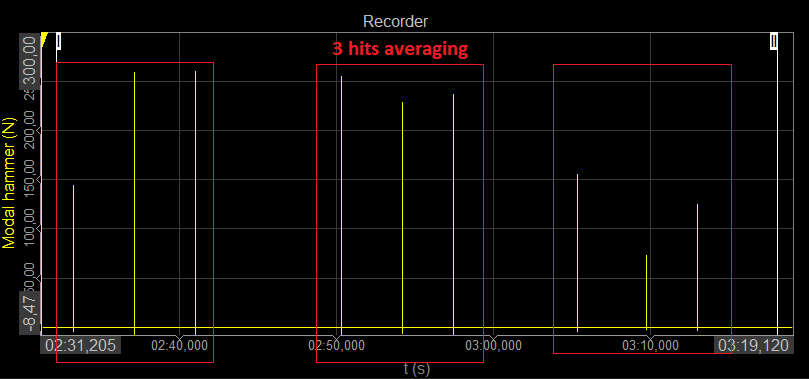### Output channels

• MIF - Mode Indicator Function is a function ranging from zero to one and shows where resonances are.
• Coherence - is a real value between zero and one. If the value is one then response power is caused totally by input power. A value less then one indicates that measured response power is greater than that from input power (noise…).
• PSD - Power Spectral Density is the measure of signal’s power content over frequency.

### Function generator

In any case, we can excite the system with help of Function generator. By checking the use function generator an additional row of configuration options is opened for shaker control or directly for input of electric circuits.

#### Impact Hammer

• Burst - Define Hold time (s)
• Chirp - Define Start and Stop frequency

#### Shaker

• Sine - Define Start and Stop frequency
• Noise - Outputs random noise

Further adjustments can be done inside of Function generator module.

### Impact hammer (Triggered)

#### Impact hammer triggering

• Pretrigger: how much data will be taken before a trigger is reached (in per cent of block size). If this is set to zero we will probably miss some data on the beginning.
• Trigger level: trigger level in units of input channel.

#### Time windows

Excitation & Response window: Define the type (Rectangular or Exponent) of the window and its length/decay in per cent. Rectangular is predefined for Excitation and Exponent for Response as this is the typical option. If your system requires you can change it.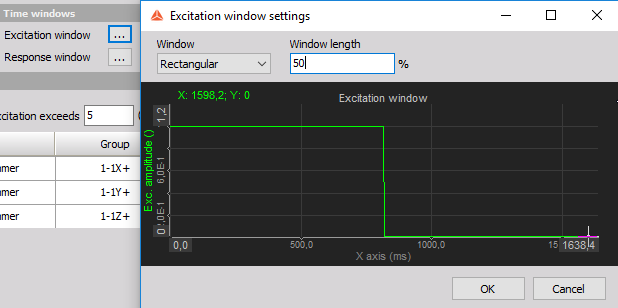#### Double hit detection

Enable or disable second hit level detection with the check box and enter the desired value in the field below. The signal will be searched for peaks and if there is more than one peak which is higher than double hit detection level you will get a warning that double hit happened. You can check it on excitation spectrum. Then you can reject this measurement or continue.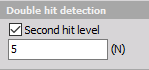### Shaker (Free run)

• FFT window - Standard Dewesoft window functions for FFT leakage reduction.
• Signal overlap - Block of data can also be overlapped.

### Operational deflection shapes (ODS)

In ODS mode settings are similar to the Shaker method, except that we can’t use the Function generator and we are limited to one Reference “excitation” channel.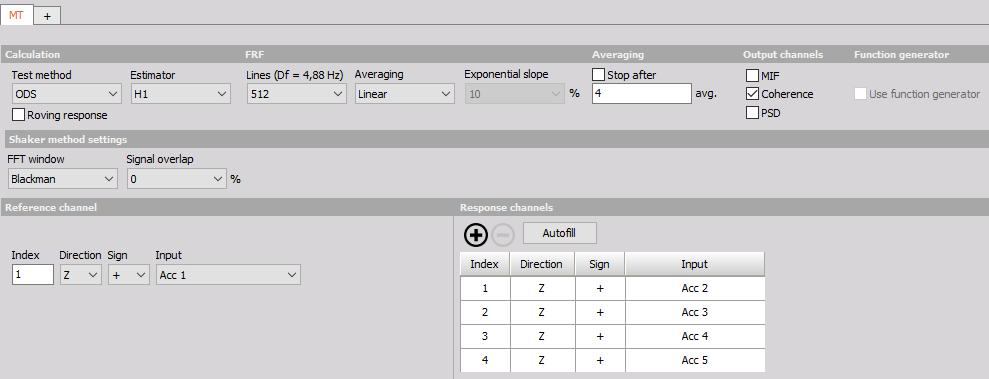### Excitation, response and reference channel settings

Show message if excitation exceeds - This is just a safety that we don’t damage our structure. When the response acceleration from our system is above the limit analogue out and measurement are stopped.

Excitation and response configurations

• Index - index of measured point (according to geometry).
• Direction - in which direction (x, y, z) we measure certain point (according to geometry).
• Sign - in which direction of axis we measure (+, -).
• Input - Input channel that is physically connected force or an acceleration sensor.
• Group - Define the measurement group when using Roving hammer/response
• AO channel - Analog Output channel which is used for driving shaker.
• Autofill - Autofill setup is used to add a large number of channels

### Autofill setup

NOTE: If you wish to use Autofill for triaxial sensors you must connect the response channels (Triaxial accelerometers) in a manner of: 1st sensor X, Y, Z, 2nd sensor X, Y, Z, and so on.

Input channels settings

AI start node input channel - Select the channel that will measure the first node of the structure Triaxial sensors (only for response ch.) - If multi-axial sensors are used, check the box to define the used channels under Geometry settings / Directions

Geometry settings

• Start node index - Define the starting point node of the structure
• Node index increment - Set the increment by which the nodes will advance
• Direction(s) - Select the direction(s) of the measurement (if Triaxial response  you can select multiple)
• Sign - Define if the excitation will be positive or negative regarding the Direction.
• Sensors in group - Define the number of sensors in one rowing group
• Roving count - Define the number of positions, how many moves of the sensor group

### Outputs

For each measured point

• Amplitude - signal amplitude.
• Phase - signal phase.
• Real - the real part of a signal.
• Imaginary - the imaginary part of a signal.
• Coherence - is a real value between zero and one. If the value is one then response power is caused totally by input power. A value less then one indicates that measured response power is greater than that from input power (noise…).

For excitations

Excitation spectrum - FFT spectrum of an excitation signal.

Common channels

• Info - information about which point is currently measured.
• Ave Count - a number of collected averages.
• Reject last - this is a channel which can be used with visual control to reject last sample (overload of channels, double hit…).
• Next point - this is a channel which can be used with visual control to go to the next point when grouping is used.

## Measure

### Visualize transfer function calculation

How to visualize transfer function calculation with FRF Geometry visual control see Geometry display.

Reject Last: in the triggered mode it is also possible to reject the last block of data if we made a false measurement. This can be done via a control button in the measurement. If we reject a block then the average count decreases by 1.

### FRF geometry

Model

• Edit

Opens UNV editor, where you can create the structure in Cartesian or Cylindrical coordinate system.

Node selection

Excitation - Select the excitation point you want to observe Response - Select the response point you want to observe Show responses in direction - Select the directions to be animated. Possible if measured in multiple axes.

View

• Coordinate system - Display or hide the X, Y, Z axes
• Nodes - Display or hide nodes / measured points
• Node numbers - Display or hide node numbers
• Lines - Show connecting lines between nodes
• Surfaces - Display or hide surfaces
• Rotate/ Scale - Switch between rotate and scale with left mouse button
• Select position - Select between different viewing positions
• Animation - Define the scale and speed of the animation or switch to manual and adjust the phase by hand.
• Frequency - Animate the structure at frequency of cursor channel or  Manually by inputting the frequency in the bottom field.
• Advanced - Lets us select the Complex, Real or Imaginary mode.

### Circle fit (exact damping estimation)

This is a visual control for basic modal analysis. You can use the yellow cursor in a 2D graph and set it to the frequency content of interest. Circle fit will find the nearest peak (resonance) and fit the circle into that data set. Then it will calculate the damping factor and more exact frequency (between line resolution of FFT).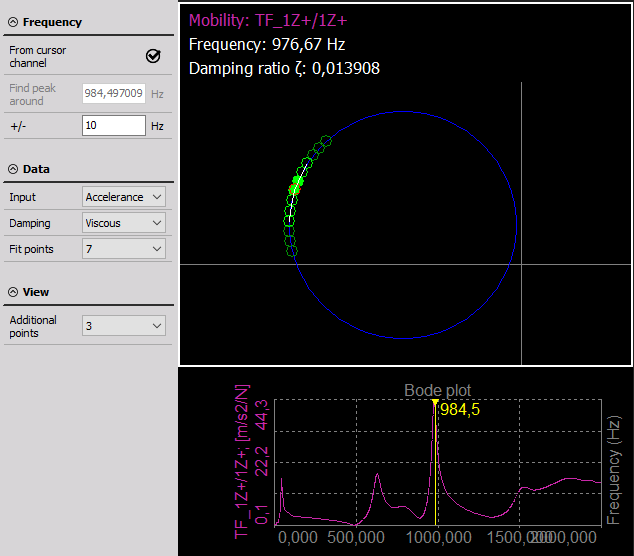• From cursor channel - the yellow cursor on the 2D graph is taken for frequency point determination.
• Manual - you can manually enter frequency point for circle fit procedure.
• Peak search (manual mode) - an area in which we will search for peak for circle fit.
• Neighbour count - a number of neighbours taken into account when doing the circle fit.